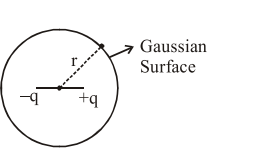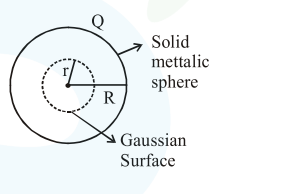# Given below are two statements :Question:

Given below are two statements :

Statement I : An electric dipole is placed at the centre of a hollow sphere. The flux of electric field through the sphere is zero but the electric field is not zero anywhere in the sphere.

Statement II : If $R$ is the radius of a solid metallic sphere and $\mathrm{Q}$ be the total charge on it. The electric field at any point on the spherical surface of radius $r( 1. Both Statement I and Statement II are true 2. Statement I is true but Statement II is false 3. Both Statement I and Statement II are false 4. Statement I is false but Statement II is true. Correct Option: , 2 Solution:$\oint \overrightarrow{\mathrm{E}} \cdot \overrightarrow{\mathrm{ds}}=\frac{\mathrm{q}_{\mathrm{in}}}{\varepsilon_{0}}=0=\phi$Flux of$\overrightarrow{\mathrm{E}}$through sphere is zero. But$\oint \overrightarrow{\mathrm{E}} \cdot \overrightarrow{\mathrm{ds}}=0 \Rightarrow\{\overrightarrow{\mathrm{E}} \cdot \overrightarrow{\mathrm{ds}} \neq 0\}$for small section$\mathrm{ds}$only Statement-2As change encloses within gaussian surface is equal to zero.$\phi=\oint \overrightarrow{\mathrm{E}} \cdot \overline{\mathrm{ds}}=0\$

Option(2) statement- 1 correct statement- 2 false.